### 13.1.2.1 A simple car

One of the easiest examples to understand is the simple car, which is shown in Figure 13.1. We all know that a car cannot drive sideways because the back wheels would have to slide instead of roll. This is why parallel parking is challenging. If all four wheels could be turned simultaneously toward the curb, it would be trivial to park a car. The complicated maneuvers for parking a simple car arise because of rolling constraints.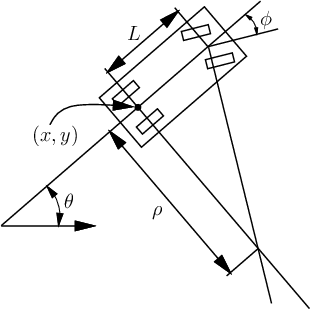The car can be imagined as a rigid body that moves in the plane. Therefore, its C-space is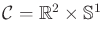. Figure 13.1 indicates several parameters associated with the car. A configuration is denoted by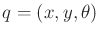. The body frame of the car places the origin at the center of rear axle, and the-axis points along the main axis of the car. Let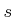denote the (signed) speed13.2 of the car. Let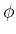denote the steering angle (it is negative for the wheel orientations shown in Figure 13.1). The distance between the front and rear axles is represented as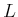. If the steering angle is fixed at, the car travels in a circular motion, in which the radius of the circle is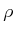. Note thatcan be determined from the intersection of the two axes shown in Figure 13.1 (the angle between these axes is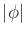).

Using the current notation, the task is to represent the motion of the car as a set of equations of the form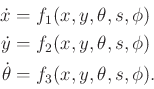(13.11)

In a small time interval,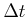, the car must move approximately in the direction that the rear wheels are pointing. In the limit astends to zero, this implies that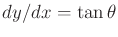. Since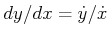and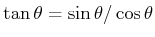, this condition can be written as a Pfaffian constraint (recall (13.5)):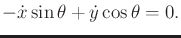(13.12)

The constraint is satisfied if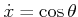and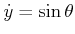. Furthermore, any scalar multiple of this solution is also a solution; the scaling factor corresponds directly to the speedof the car. Thus, the first two scalar components of the configuration transition equation are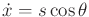and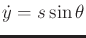.

The next task is to derive the equation for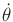. Let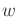denote the distance traveled by the car (the integral of speed). As shown in Figure 13.1,represents the radius of a circle that is traversed by the center of the rear axle, if the steering angle is fixed. Note that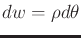. From trigonometry,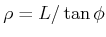, which implies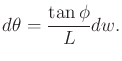(13.13)

Dividing both sides by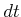and using the fact that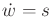yields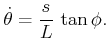(13.14)

So far, the motion of the car has been modeled, but no action variables have been specified. Suppose that the speedand steering angleare directly specified by the action variables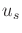and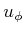, respectively. The convention of using avariable with the old variable name appearing as a subscript will be followed. This makes it easy to identify the actions in a configuration transition equation. A two-dimensional action vector,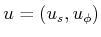, is obtained. The configuration transition equation for the simple car is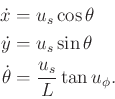(13.15)

As expressed in (13.15), the transition equation is not yet complete without specifying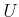, the set of actions of the form. First suppose that any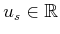is possible. What steering angles are possible? The interval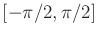is sufficiently large for the steering anglebecause any other value is equivalent to one between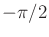and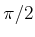. Steering angles ofandare problematic. To derive the expressions for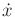and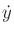, it was assumed that the car moves in the direction that the rear wheels are pointing. Imagine you are sitting on a tricycle and turn the front wheel perpendicular to the rear wheels (assigning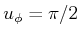). If you are able to pedal, then the tricycle should rotate in place. This means that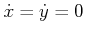because the center of the rear axle does not translate.

This strange behavior is not allowed for a standard automobile. A car with rear-wheel drive would probably skid the front wheels across the pavement. If a car with front-wheel drive attempted this, it should behave as a tricycle; however, this is usually not possible because the front wheels would collide with the front axle when turned to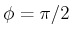. Therefore, the simple car should have a maximum steering angle,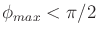, and we require that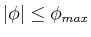. Observe from Figure 13.1 that a maximum steering angle implies a minimum turning radius,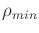. For the case of a tricycle,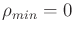. You may have encountered the problem of a minimum turning radius while trying to make an illegal U-turn. It is sometimes difficult to turn a car around without driving it off of the road.

Now return to the speed. On level pavement, a real vehicle has a top speed, and its behavior should change dramatically depending on the speed. For example, if you want to drive along the minimum turning radius, you should not drive at 140km/hr. It seems that the maximum steering angle should reduce at higher speeds. This enters the realm of dynamics, which will be allowed after phase spaces are introduced in Section 13.2. Following this, some models of cars with dynamics will be covered in Sections 13.2.4 and 13.3.3.

It has been assumed implicitly that the simple car is moving slowly to safely neglect dynamics. A bound such as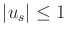can be placed on the speed without affecting the configurations that it can reach. The speed can even be constrained as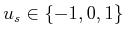without destroying reachability. Be careful, however, about a bound such as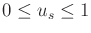. In this case, the car cannot drive in reverse! This clearly affects the set of reachable configurations. Imagine a car that is facing a wall and is unable to move in reverse. It may be forced to hit the wall as it moves.

Based on these considerations regarding the speed and steering angle, several interesting variations are possible:

1. [] Tricycle: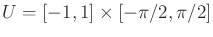. Assuming front-wheel drive, the car'' can rotate in place ifor. This is unrealistic for a simple car. The resulting model is similar to that of the simple unicycle, which appears later in (13.18).
2. [] Simple Car :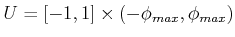. By requiring that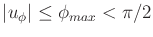, a car with minimum turning radius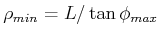is obtained.
3. [] Reeds-Shepp Car [814,923]: Further restrict the speed of the simple car so that.13.3 This model intuitively makescorrespond to three discrete gears'': reverse, park, or forward. An interesting question under this model is: What is the shortest possible path (traversed in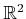by the center of the rear axle) between two configurations in the absence of obstacles? This is answered in Section 15.3.
4. [] Dubins Car : Remove the reverse speed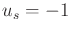from the Reeds-Shepp car to obtain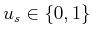as the only possible speeds. The shortest paths infor this car are quite different than for the Reeds-Shepp car; see Section 15.3.
The car that was shown in Figure 1.12a of Section 1.2 is even more restricted than the Dubins car because it is additionally forced to turn left.

Basic controllability issues have been studied thoroughly for the simple car. These will be covered in Section 15.4, but it is helpful to develop intuitive notions here to assist in understanding the planning algorithms of Chapter 14. The simple car is considered nonholonomic because there are differential constraints that cannot be completely integrated. This means that the car configurations are not restricted to a lower dimensional subspace of. The Reeds-Shepp car can be maneuvered into an arbitrarily small parking space, provided that a small amount of clearance exists. This property is called small-time local controllability and is presented in Section 15.1.3. The Dubins car is nonholonomic, but it does not possess this property. Imagine the difficulty of parallel parking without using the reverse gear. In an infinitely large parking lot without obstacles, however, the Dubins car can reach any configuration.

Steven M LaValle 2020-08-14